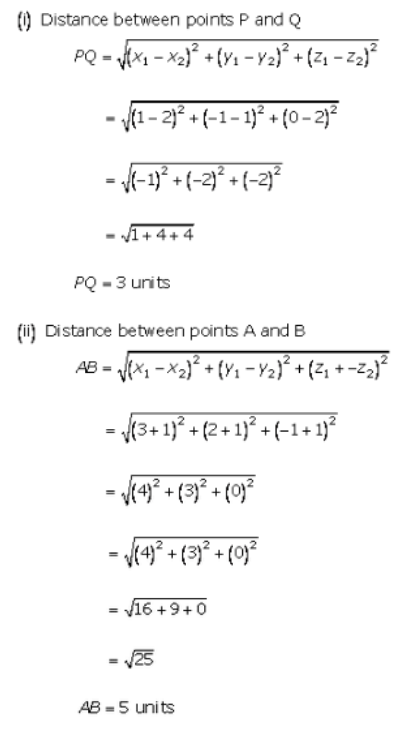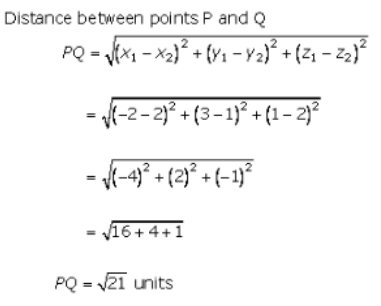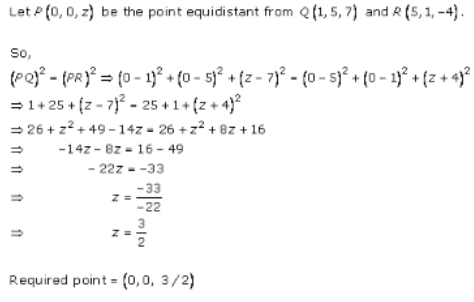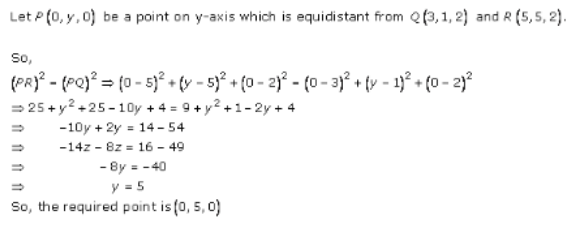×#### Thank you for registering.

One of our academic counsellors will contact you within 1 working day.

Click to Chat

1800-1023-196

+91-120-4616500

CART 0

• 0

MY CART (5)

Use Coupon: CART20 and get 20% off on all online Study Material

ITEM
DETAILS
MRP
DISCOUNT
FINAL PRICE
Total Price: Rs.

There are no items in this cart.
Continue Shopping• Complete JEE Main/Advanced Course and Test Series
• OFFERED PRICE: Rs. 15,900
• View Details

```Chapter 28: Introduction to 3D coordinate geometry – Exercise 28.2

Introduction to 3D coordinate geometry – Exercise – 28.2 – Q.1Introduction to 3D coordinate geometry – Exercise – 28.2 – Q.2Introduction to 3D coordinate geometry – Exercise – 28.2 – Q.3(i)Introduction to 3D coordinate geometry – Exercise – 28.2 – Q.3(ii)Introduction to 3D coordinate geometry – Exercise – 28.2 – Q.3(iii)Introduction to 3D coordinate geometry – Exercise – 28.2 – Q.4(i)Introduction to 3D coordinate geometry – Exercise – 28.2 – Q.4(ii)Introduction to 3D coordinate geometry – Exercise – 28.2 – Q.4(iii)Introduction to 3D coordinate geometry – Exercise – 28.2 – Q.5Introduction to 3D coordinate geometry – Exercise – 28.2 – Q.6```### Course Features

• 728 Video Lectures
• Revision Notes
• Previous Year Papers
• Mind Map
• Study Planner
• NCERT Solutions
• Discussion Forum
• Test paper with Video Solution